Prioritized Categories All categories Not categorized G-C G-CO G-GMD G-GPE G-MD G-SRT Modeling Prioritized

Page:  1  2  3  (Next)
ALL

#### MAT-HS.G-CO.09

 MAT-HS Targeted Standards(G) Concept: Geometry(CO) Domain: CongruenceCluster: Prove geometric theorems MAT-HS.G-CO.09 Prove and apply theorems about lines and angles. Theorems include: vertical angles are congruent; when a transversal crosses parallel lines, alternate interior angles are congruent and corresponding angles are congruent; points on a perpendicular bisector of a line segment are exactly those equidistant from the segment’s endpoints.

## Student Learning Targets:

• I can
• I can

• I can
• I can

### Skills (Performance) Targets

• I can prove theorems pertaining to lines and angles.
• I can prove vertical angles are congruent.
• I can prove when a transversal crosses parallel lines, alternate interior angles are congruent and corresponding angles are congruent.
• I can prove points on a perpendicular bisector of a line segment are exactly those equidistant from the segment’s endpoints.

• I can
• I can

## Proficiency Scale

 Score Description Sample Activity 4.0 In addition to Score 3.0, the student demonstrates in-depth inferences and applications regarding more complex material that go beyond end of instruction expectations. - 3.5 In addition to Score 3.0 performance, the student demonstrates in-depth inferences and applications regarding the more complex content with partial success. 3.0 “The Standard.” The student demonstrates no major errors or omissions regarding any of the information and processes that were end of instruction expectations. - 2.5 The student demonstrates no major errors or omissions regarding the simpler details and processes (Score 2.0 content) and partial knowledge of the more complex ideas and processes (Score 3.0 content). 2.0 The student demonstrates no major errors or omissions regarding the simpler details and processes but exhibits major errors or omissions regarding the more complex ideas and processes (Score 3.0 content). - 1.5 The student demonstrates partial knowledge of the simpler details and processes (Score 2.0 content) but exhibits major errors or omissions regarding the more complex ideas and procedures (Score 3.0 content). 1.0 With help, the student demonstrates a partial understanding of some of the simpler details and processes (Score 2.0 content) and some of the more complex ideas and processes (Score 3.0 content). - 0.5 With help, the student demonstrates a partial understanding of some of the simpler details and processes (Score 2.0 content) but not the more complex ideas and processes (Score 3.0 content). 0.0 Even with help, the student demonstrates no understanding or skill. -

## Resources

 Web Vocab

#### MAT-HS.G-CO.09.a

 MAT-HS Targeted Standards(G) Concept: Geometry(CO) Domain: CongruenceCluster: Prove geometric theorems MAT-HS.G-CO.09.a Prove theorems about lines and angles. Theorems include but are not limited to: vertical angles are congruent; when a transversal crosses parallel lines, alternate interior angles are congruent and corresponding angles are congruent; points on a perpendicular bisector of a line segment are exactly those equidistant from the segment’s endpoints.

• I can
• I can

• I can
• I can

• I can
• I can

• I can
• I can

## Proficiency Scale

 Score Description Sample Activity Advanced In addition to Score 3.0, the student demonstrates in-depth inferences and applications regarding more complex material that go beyond end of instruction expectations. Error analysis (find errors in a given proof, fix the error and explain why it was incorrect) Concepts: Vertical Angles Angles created by parallel lines and a transversal Perpendicular Bisector theorems - 3.5 In addition to Score 3.0 performance, the student demonstrates in-depth inferences and applications regarding the more complex content with partial success. Proficient “The Standard.” The student demonstrates no major errors or omissions regarding any of the information and processes that were end of instruction expectations. Identify relevant given information Arrange reasoning in a logical sequence Support reasoning using appropriate justifications (theorems, postulates, definitions, etc.) Concepts: Vertical Angles Angles created by parallel lines and a transversal Perpendicular Bisector theorems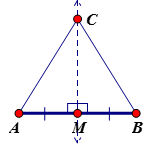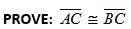2.5 The student demonstrates no major errors or omissions regarding the simpler details and processes (Score 2.0 content) and partial knowledge of the more complex ideas and processes (Score 3.0 content). Progressing The student demonstrates no major errors or omissions regarding the simpler details and processes but exhibits major errors or omissions regarding the more complex ideas and processes (Score 3.0 content). partially prove but reasoning has gaps, steps missing, incorrect reasoning, etc. recall theorems, definitions, and postulates fill out justification given a statement Concepts: Vertical Angles Angles created by parallel lines and a transversal Perpendicular Bisector theorems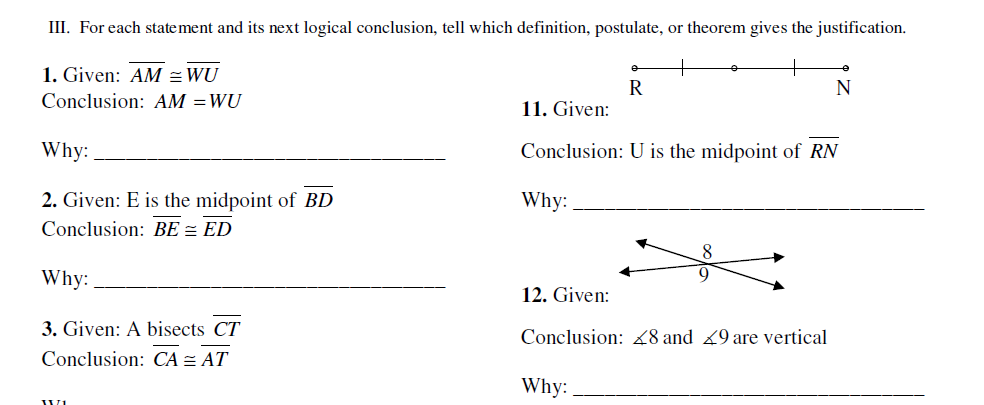1.5 The student demonstrates partial knowledge of the simpler details and processes (Score 2.0 content) but exhibits major errors or omissions regarding the more complex ideas and procedures (Score 3.0 content). Beginning With help, the student demonstrates a partial understanding of some of the simpler details and processes (Score 2.0 content) and some of the more complex ideas and processes (Score 3.0 content). - 0.5 With help, the student demonstrates a partial understanding of some of the simpler details and processes (Score 2.0 content) but not the more complex ideas and processes (Score 3.0 content). 0.0 Even with help, the student demonstrates no understanding or skill. -

## Resources

 Web Vocab

#### MAT-HS.G-CO.09.b

 MAT-HS Targeted Standards(G) Concept: Geometry(CO) Domain: CongruenceCluster: Prove geometric theorems MAT-HS.G-CO.09.b Apply theorems about lines and angles. Theorems include: vertical angles are congruent; when a transversal crosses parallel lines, alternate interior angles are congruent and corresponding angles are congruent; points on a perpendicular bisector of a line segment are exactly those equidistant from the segment’s endpoints. (Focus is on applying the theorems for C-CO.9B)

• I can
• I can

• I can
• I can

• I can
• I can

• I can
• I can

## Proficiency Scale

 Score Description Sample Activity Advanced In addition to Score 3.0, the student demonstrates in-depth inferences and applications regarding more complex material that go beyond end of instruction expectations. Concepts: Vertical Angles Angles created by parallel lines and a transversal Perpendicular Bisector theorems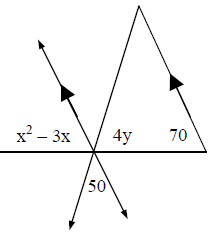Solve for x and y. 3.5 In addition to Score 3.0 performance, the student demonstrates in-depth inferences and applications regarding the more complex content with partial success. Proficient “The Standard.” The student demonstrates no major errors or omissions regarding any of the information and processes that were end of instruction expectations. Identify the relevant information Apply geometric relationships to create an algebraic model for the situation Solve and answer the given question (Ex. Solve for the given variable, Find the angle measurement) Concepts: Vertical Angles Angles created by parallel lines and a transversal Perpendicular Bisector theorem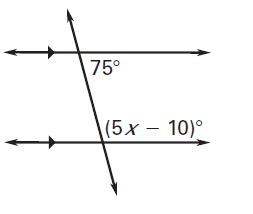Solve for x.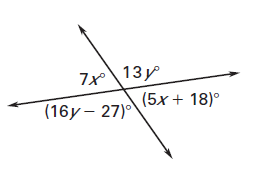Solve for x and y. 2.5 The student demonstrates no major errors or omissions regarding the simpler details and processes (Score 2.0 content) and partial knowledge of the more complex ideas and processes (Score 3.0 content). Progressing The student demonstrates no major errors or omissions regarding the simpler details and processes but exhibits major errors or omissions regarding the more complex ideas and processes (Score 3.0 content). can recognize and recall angle pairs can apply postulates, definitions, properties, and theorems to identify angle measurements Concepts: Vertical Angles Angles created by parallel lines and a transversal Perpendicular Bisector theorems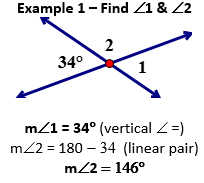Example 2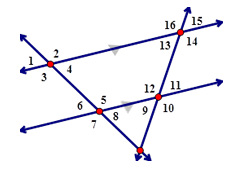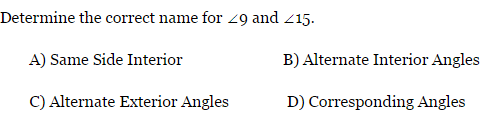1.5 The student demonstrates partial knowledge of the simpler details and processes (Score 2.0 content) but exhibits major errors or omissions regarding the more complex ideas and procedures (Score 3.0 content). Beginning With help, the student demonstrates a partial understanding of some of the simpler details and processes (Score 2.0 content) and some of the more complex ideas and processes (Score 3.0 content). - 0.5 With help, the student demonstrates a partial understanding of some of the simpler details and processes (Score 2.0 content) but not the more complex ideas and processes (Score 3.0 content). 0.0 Even with help, the student demonstrates no understanding or skill. -

## Resources

 Web Vocab

#### MAT-HS.G-CO.10

 MAT-HS Targeted Standards(G) Concept: Geometry(CO) Domain: CongruenceCluster: Prove geometric theorems MAT-HS.G-CO.10 Prove and apply theorems about triangles. Theorems include: measures of interior angles of a triangle sum to 180°; base angles of isosceles triangles are congruent; the segment joining midpoints of two sides of a triangle is parallel to the third side and half the length; the medians of a triangle meet at a point.

## Student Learning Targets:

• I can
• I can

• I can
• I can

### Skills (Performance) Targets

• I can prove theorems about triangles.
• I can prove the measures of interior angles of a triangle have a sum of 180°.
• I can prove base angles of isosceles triangles are congruent.
• I can prove the segment joining midpoints of two sides of a triangle is parallel to the third side and half the length.
• I can prove the medians of a triangle meet at a point.

• I can
• I can

## Proficiency Scale

 Score Description Sample Activity 4.0 In addition to Score 3.0, the student demonstrates in-depth inferences and applications regarding more complex material that go beyond end of instruction expectations. - 3.5 In addition to Score 3.0 performance, the student demonstrates in-depth inferences and applications regarding the more complex content with partial success. 3.0 “The Standard.” The student demonstrates no major errors or omissions regarding any of the information and processes that were end of instruction expectations. - 2.5 The student demonstrates no major errors or omissions regarding the simpler details and processes (Score 2.0 content) and partial knowledge of the more complex ideas and processes (Score 3.0 content). 2.0 The student demonstrates no major errors or omissions regarding the simpler details and processes but exhibits major errors or omissions regarding the more complex ideas and processes (Score 3.0 content). - 1.5 The student demonstrates partial knowledge of the simpler details and processes (Score 2.0 content) but exhibits major errors or omissions regarding the more complex ideas and procedures (Score 3.0 content). 1.0 With help, the student demonstrates a partial understanding of some of the simpler details and processes (Score 2.0 content) and some of the more complex ideas and processes (Score 3.0 content). - 0.5 With help, the student demonstrates a partial understanding of some of the simpler details and processes (Score 2.0 content) but not the more complex ideas and processes (Score 3.0 content). 0.0 Even with help, the student demonstrates no understanding or skill. -

## Resources

 Web Vocab

#### MAT-HS.G-CO.10.a

 MAT-HS Targeted Standards(G) Concept: Geometry(CO) Domain: CongruenceCluster: Prove geometric theorems MAT-HS.G-CO.10.a Prove theorems about triangles. (Theorems include but are not limited to: Measures of interior angles of a triangle sum to 180 degrees; base angles of isosceles triangles are congruent; the segment joining midpoints of two sides of a triangle is parallel to the third side and half the length; the medians of a triangle meet at a point.) (Focus on proving for this proficiency scale)

• I can
• I can

• I can
• I can

• I can
• I can

• I can
• I can

## Proficiency Scale

 Score Description Sample Activity Advanced In addition to Score 3.0, the student demonstrates in-depth inferences and applications regarding more complex material that go beyond end of instruction expectations. Analyze a proof with errors and make corrections - 3.5 In addition to Score 3.0 performance, the student demonstrates in-depth inferences and applications regarding the more complex content with partial success. Proficient “The Standard.” The student demonstrates no major errors or omissions regarding any of the information and processes that were end of instruction expectations.   Identify relevant given information Arrange reasoning in a logical sequence Support reasoning using appropriate justifications (theorems, postulates, definitions, etc.) Midsegment of a Triangle Proof Triangle Interior Angle Proof Prove a Triangle is Isosceles 2.5 The student demonstrates no major errors or omissions regarding the simpler details and processes (Score 2.0 content) and partial knowledge of the more complex ideas and processes (Score 3.0 content). Progressing The student demonstrates no major errors or omissions regarding the simpler details and processes but exhibits major errors or omissions regarding the more complex ideas and processes (Score 3.0 content).   partially prove but reasoning has gaps, steps missing, incorrect reasoning, etc. recall theorems, definitions, and postulates fill out justification given a statement Given a statement, the student can fill out the justification.  (skeleton proof) 1.5 The student demonstrates partial knowledge of the simpler details and processes (Score 2.0 content) but exhibits major errors or omissions regarding the more complex ideas and procedures (Score 3.0 content). Beginning With help, the student demonstrates a partial understanding of some of the simpler details and processes (Score 2.0 content) and some of the more complex ideas and processes (Score 3.0 content). - 0.5 With help, the student demonstrates a partial understanding of some of the simpler details and processes (Score 2.0 content) but not the more complex ideas and processes (Score 3.0 content). 0.0 Even with help, the student demonstrates no understanding or skill. -

## Resources

 Web Vocab

#### MAT-HS.G-CO.10.b

 MAT-HS Targeted Standards(G) Concept: Geometry(CO) Domain: CongruenceCluster: Prove geometric theorems MAT-HS.G-CO.10.b Apply theorems about triangles. (Theorems include: measures of interior angles of a triangle sum to 180 degrees; base angles of isosceles triangles are congruent; the segment joining midpoints of two sides of a triangle is parallel to the third side and half the length; the medians of a triangle meet at a point. (Focus on Applying)

• I can
• I can

• I can
• I can

• I can
• I can

• I can
• I can

## Proficiency Scale

 Score Description Sample Activity Advanced In addition to Score 3.0, the student demonstrates in-depth inferences and applications regarding more complex material that go beyond end of instruction expectations. Analyze a solution and correct the errors Investigate and provide multiple methods for finding a solution to a given problem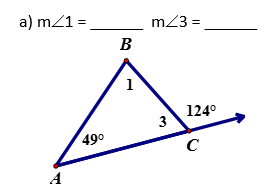Find the measures of these angles in two different ways. Explain the steps you took for each method. 3.5 In addition to Score 3.0 performance, the student demonstrates in-depth inferences and applications regarding the more complex content with partial success. Proficient “The Standard.” The student demonstrates no major errors or omissions regarding any of the information and processes that were end of instruction expectations. Apply theorems (postulates, definitions, etc.) about triangles to solve problems Justify how to set up a problem using theorems (postulates, definitions, etc.) about triangles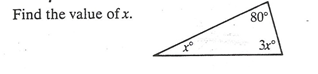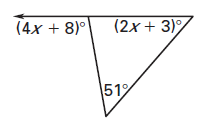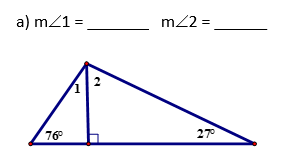2.5 The student demonstrates no major errors or omissions regarding the simpler details and processes (Score 2.0 content) and partial knowledge of the more complex ideas and processes (Score 3.0 content). Progressing The student demonstrates no major errors or omissions regarding the simpler details and processes but exhibits major errors or omissions regarding the more complex ideas and processes (Score 3.0 content).   Identify or relate the theorem used to set up the problem Define the vocabulary associated with triangle theorems Can identify the components of triangles - 1.5 The student demonstrates partial knowledge of the simpler details and processes (Score 2.0 content) but exhibits major errors or omissions regarding the more complex ideas and procedures (Score 3.0 content). Beginning With help, the student demonstrates a partial understanding of some of the simpler details and processes (Score 2.0 content) and some of the more complex ideas and processes (Score 3.0 content). - 0.5 With help, the student demonstrates a partial understanding of some of the simpler details and processes (Score 2.0 content) but not the more complex ideas and processes (Score 3.0 content). 0.0 Even with help, the student demonstrates no understanding or skill. -

## Resources

 Web Vocab

#### MAT-HS.G-CO.11

 MAT-HS Targeted Standards(G) Concept: Geometry(CO) Domain: CongruenceCluster: Prove geometric theorems MAT-HS.G-CO.11 Prove and apply theorems about parallelograms. Theorems include: opposite sides are congruent, opposite angles are congruent, the diagonals of a parallelogram bisect each other, and conversely, rectangles are parallelograms with congruent diagonals.

## Student Learning Targets:

• I can
• I can

• I can
• I can

### Skills (Performance) Targets

• I can prove theorems about parallelograms.
• I can prove opposite sides are congruent.
• I can prove opposite angles are congruent.
• I can prove the diagonals of a parallelogram bisect each other.
• I can prove rectangles are parallelograms with congruent diagonals.

• I can
• I can

## Proficiency Scale

 Score Description Sample Activity 4.0 In addition to Score 3.0, the student demonstrates in-depth inferences and applications regarding more complex material that go beyond end of instruction expectations. - 3.5 In addition to Score 3.0 performance, the student demonstrates in-depth inferences and applications regarding the more complex content with partial success. 3.0 “The Standard.” The student demonstrates no major errors or omissions regarding any of the information and processes that were end of instruction expectations. - 2.5 The student demonstrates no major errors or omissions regarding the simpler details and processes (Score 2.0 content) and partial knowledge of the more complex ideas and processes (Score 3.0 content). 2.0 The student demonstrates no major errors or omissions regarding the simpler details and processes but exhibits major errors or omissions regarding the more complex ideas and processes (Score 3.0 content). - 1.5 The student demonstrates partial knowledge of the simpler details and processes (Score 2.0 content) but exhibits major errors or omissions regarding the more complex ideas and procedures (Score 3.0 content). 1.0 With help, the student demonstrates a partial understanding of some of the simpler details and processes (Score 2.0 content) and some of the more complex ideas and processes (Score 3.0 content). - 0.5 With help, the student demonstrates a partial understanding of some of the simpler details and processes (Score 2.0 content) but not the more complex ideas and processes (Score 3.0 content). 0.0 Even with help, the student demonstrates no understanding or skill. -

## Resources

 Web Vocab

#### MAT-HS.G-CO.11.a

 MAT-HS Targeted Standards(G) Concept: Geometry(CO) Domain: CongruenceCluster: Prove geometric theorems MAT-HS.G-CO.11.b Prove theorems about parallelograms. (Focus on Prove for G-CO.11) (Theorems include but are not limited to: opposite sides are congruent, opposite angles are congruent, the diagonals of a parallelogram bisect each other, and conversely, rectangles are parallelograms with congruent diagonals.)

• I can
• I can

• I can
• I can

• I can
• I can

• I can
• I can

## Proficiency Scale

 Score Description Sample Activity Advanced In addition to Score 3.0, the student demonstrates in-depth inferences and applications regarding more complex material that go beyond end of instruction expectations. Analyze a proof with errors and make corrections - 3.5 In addition to Score 3.0 performance, the student demonstrates in-depth inferences and applications regarding the more complex content with partial success. Proficient “The Standard.” The student demonstrates no major errors or omissions regarding any of the information and processes that were end of instruction expectations. The student can perform all the actions for each concept listed below: Identify relevant information Arrange reasoning in a logical sequence Support reasoning using appropriate justifications (theorems, postulates, definitions, etc.)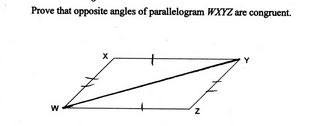2.5 The student demonstrates no major errors or omissions regarding the simpler details and processes (Score 2.0 content) and partial knowledge of the more complex ideas and processes (Score 3.0 content). Progressing The student demonstrates no major errors or omissions regarding the simpler details and processes but exhibits major errors or omissions regarding the more complex ideas and processes (Score 3.0 content). Partially prove but reasoning has gaps, steps missing, incorrect reasoning, etc. Recall theorems, definitions, and postulates Fill out justification given a statement - 1.5 The student demonstrates partial knowledge of the simpler details and processes (Score 2.0 content) but exhibits major errors or omissions regarding the more complex ideas and procedures (Score 3.0 content). Beginning With help, the student demonstrates a partial understanding of some of the simpler details and processes (Score 2.0 content) and some of the more complex ideas and processes (Score 3.0 content). - 0.5 With help, the student demonstrates a partial understanding of some of the simpler details and processes (Score 2.0 content) but not the more complex ideas and processes (Score 3.0 content). 0.0 Even with help, the student demonstrates no understanding or skill. -

## Resources

 Web Vocab

#### MAT-HS.G-CO.11.b

 MAT-HS Targeted Standards(G) Concept: Geometry(CO) Domain: CongruenceCluster: Prove geometric theorems MAT-HS.G-CO.11.b Apply theorems about parallelograms. Theorems include but are not limited to: opposite sides are congruent, opposite angles are congruent, the diagonals of a parallelogram bisect each other, and conversely, rectangles are parallelograms with congruent diagonals. (Focus is on Application for G-CO.11B)

• I can
• I can

• I can
• I can

• I can
• I can

• I can
• I can

## Proficiency Scale

 Score Description Sample Activity Advanced In addition to Score 3.0, the student demonstrates in-depth inferences and applications regarding more complex material that go beyond end of instruction expectations. - 3.5 In addition to Score 3.0 performance, the student demonstrates in-depth inferences and applications regarding the more complex content with partial success. Proficient “The Standard.” The student demonstrates no major errors or omissions regarding any of the information and processes that were end of instruction expectations. Apply theorems (postulates, definitions, etc.) about parallelograms to solve problems Justify how to set up a problem using theorems (postulates, definitions, etc.) about parallelograms Solve for x and y.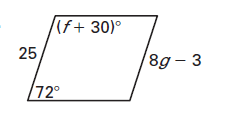2.5 The student demonstrates no major errors or omissions regarding the simpler details and processes (Score 2.0 content) and partial knowledge of the more complex ideas and processes (Score 3.0 content). Progressing The student demonstrates no major errors or omissions regarding the simpler details and processes but exhibits major errors or omissions regarding the more complex ideas and processes (Score 3.0 content). Identify the theorem, postulate, or definition used to set up and solve a problem Able to recognize and recall important vocabulary such as:                 opposite sides                 opposite angles                 diagonals                 congruent triangles                  perpendicular                 CPCTC Students are able to find the missing measurements  in a parallelogram given some basic information Can determine if a given figure is a parallelogram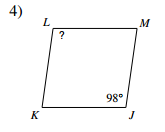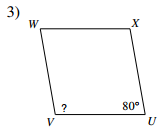Identify if the given figure is a parallelogram.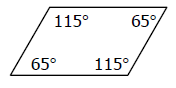1.5 The student demonstrates partial knowledge of the simpler details and processes (Score 2.0 content) but exhibits major errors or omissions regarding the more complex ideas and procedures (Score 3.0 content). Beginning With help, the student demonstrates a partial understanding of some of the simpler details and processes (Score 2.0 content) and some of the more complex ideas and processes (Score 3.0 content). - 0.5 With help, the student demonstrates a partial understanding of some of the simpler details and processes (Score 2.0 content) but not the more complex ideas and processes (Score 3.0 content). 0.0 Even with help, the student demonstrates no understanding or skill. -

## Resources

 Web Vocab

#### MAT-HS.G-CO.12

 MAT-HS Targeted Standards(G) Concept: Geometry(CO) Domain: CongruenceCluster: Make geometric constructions MAT-HS.G-CO.12 Make basic geometric constructions with a variety of tools and methods. Copying a segment; copying an angle; bisecting a segment; bisecting an angle; constructing perpendicular lines, including the perpendicular bisector of a line segment; and constructing a line parallel to a given line through a point not on the line.  Tools may include compass and straightedge, string, reflective devices, paper folding or dynamic geometric software.

## Student Learning Targets:

• I can
• I can

• I can
• I can

### Skills (Performance) Targets

• I can use a variety of methods and tools to:
• copy a segment
• copy an angle
• bisect a segment
• bisect an angle
• construct perpendicular
• lines, including the perpendicular bisector of a line segment
• construct a line parallel to a given line through a point not on the line

• I can
• I can

## Proficiency Scale

 Score Description Sample Activity Advanced In addition to Score 3.0, the student demonstrates in-depth inferences and applications regarding more complex material that go beyond end of instruction expectations. Explain how to construct a geometric figure Explain the type of construction and the next step in completing the construction, giving specific steps.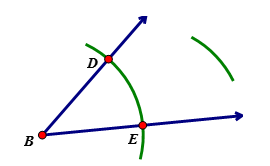3.5 In addition to Score 3.0 performance, the student demonstrates in-depth inferences and applications regarding the more complex content with partial success. Proficient “The Standard.” The student demonstrates no major errors or omissions regarding any of the information and processes that were end of instruction expectations. Construct a geometric figure using various tools and/or methods  (Ex: compass and straightedge, Geogebra) (a) copy a given line segment   (b) copy an angle. (c) bisect a line segment. (d) bisect an angle. (e) construct a line perpendicular to a given line through a point on that line. (f) construct a line perpendicular to a given line through a point not on that line. (g) construct the perpendicular bisector of a line segment. (h) construct a line parallel to a given line through a point not on the line.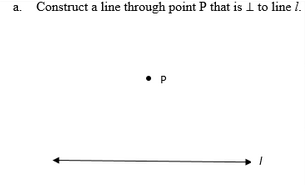Identify and complete the construction.2.5 The student demonstrates no major errors or omissions regarding the simpler details and processes (Score 2.0 content) and partial knowledge of the more complex ideas and processes (Score 3.0 content). Progressing The student demonstrates no major errors or omissions regarding the simpler details and processes but exhibits major errors or omissions regarding the more complex ideas and processes (Score 3.0 content). can recognize and recall terminology to answer questions  identify the type of construction given an example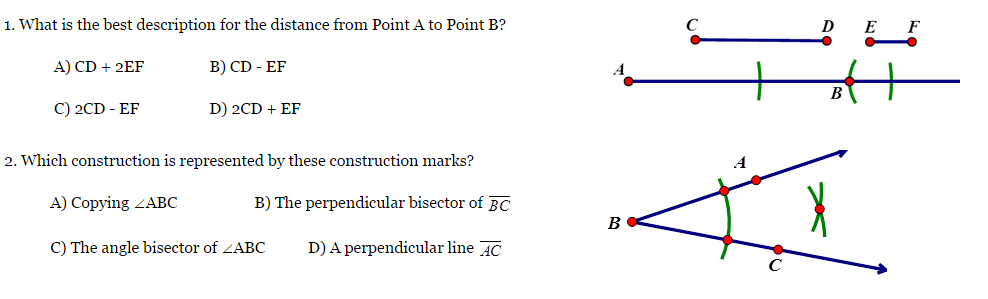1.5 The student demonstrates partial knowledge of the simpler details and processes (Score 2.0 content) but exhibits major errors or omissions regarding the more complex ideas and procedures (Score 3.0 content). Beginning With help, the student demonstrates a partial understanding of some of the simpler details and processes (Score 2.0 content) and some of the more complex ideas and processes (Score 3.0 content). - 0.5 With help, the student demonstrates a partial understanding of some of the simpler details and processes (Score 2.0 content) but not the more complex ideas and processes (Score 3.0 content). 0.0 Even with help, the student demonstrates no understanding or skill. -

## Resources

 Web Vocab

Page:  1  2  3  (Next)
ALL